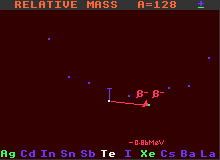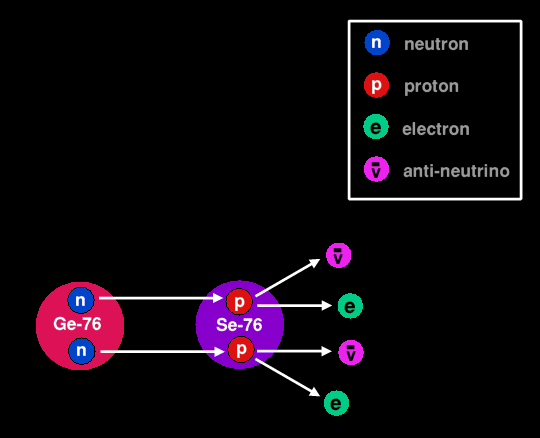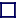### DOUBLE BETA DECAY

Some nuclides with neutron excesses, although unstable, are forbidden to decay by single beta decay because the immediate product has greater mass and instead must decay by double beta decay, where both occur simultaneously. This event is doubly improbable, hence such nuclides have very long half-lives.A=128 Mass Diagram for the double-beta decay of Tellurium-128

Double beta decay can only occur in nuclei that contain EVEN numbers of both protons and neutrons. Some of these so called 'even-even' nuclei cannot decay by normal beta decay because the resulting nucleus would be heavier than the original nucleus, and is thus forbidden by conservation of energy. But by changing TWO neutrons simultaneously into protons, these even-even nuclei can surmount the intervening energy barrier and reach nuclei that are lighter, so this double beta decay should be allowed. But since beta decay involves the improbable action of the weak force, these double beta decays are doubly improbable, and hence highly unlikely to occur often. This gives rise to nuclides with enormously long half-lives, many trillions of times longer than the present age of the Universe, which is only about 13,700 million years old.Normal Double Beta Decay of Germanium-76
Two neutrons simultaneously decay into two protons plus two electrons and two anti-neutrinos

Examples of nuclides decaying by double beta decay are tellurium-130 which becomes xenon-130; selenium-82 which becomes krypton-82; and germanium-76 which becomes selenium-76. Tellurium-128 has a half-life of 5×1024 years. It is still debated whether double beta decay involves the emission of two similar neutrinos, or of a particle/anti-particle pair of neutrinos which annihilate each other with consequent no net neutrino emission.

There are 40 nuclides that are theoretically capable of double beta decay, but with the huge improbability of double beta decay, not all have actually been observed to do so.

Shown is tellurium-128 decaying by double beta decay into stable xenon-128